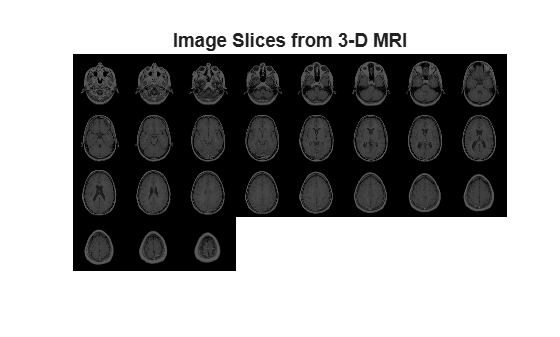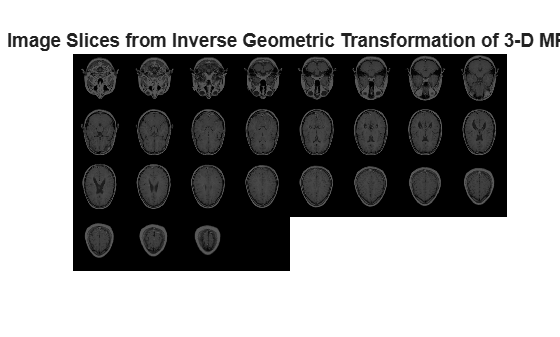# geometricTransform3d

3-D geometric transformation object

## Description

A `geometricTransform3d` object defines a custom 3-D geometric transformation using point-wise mapping functions.

## Creation

### Syntax

``tform = geometricTransform3d(inverseFcn)``
``tform = geometricTransform3d(inverseFcn,forwardFcn)``

### Description

example

````tform = geometricTransform3d(inverseFcn)` creates a `geometricTransform3d` object and sets the value of inverse mapping function property, `InverseFcn` to `inverseFcn`.```
````tform = geometricTransform3d(inverseFcn,forwardFcn)` also sets the value of forward mapping function property, `ForwardFcn` to `forwardFcn`.```

## Properties

expand all

Inverse mapping function, specified as a function handle. The function must accept and return coordinates as an n-by-3 numeric matrix representing the packed (x,y,z) coordinates of n points.

Example: `ifcn = @(xyz) [xyz(:,1).^2,xyz(:,2).^2,xyz(:,3).^2];`

Forward mapping function, specified as a function handle. The function must accept and return coordinates as an n-by-3 numeric matrix representing the packed (x,y,z) coordinates of n points.

Example: ```ffcn = @(xyz) [sqrt(xyz(:,1)),sqrt(xyz(:,2)),sqrt(xyz(:,3))];```

## Object Functions

 `transformPointsForward` Apply forward geometric transformation `transformPointsInverse` Apply inverse geometric transformation

## Examples

collapse all

Specify the packed (x,y,z) coordinates of five input points. The packed coordinates are stored as a 5-by-3 matrix, where the first, second, and third columns contain the x-, y-, and z- coordinates,respectively.

`XYZ = [5 25 20;10 5 25;15 10 5;20 15 10;25 20 15];`

Define an inverse mapping function that accepts and returns points in packed (x,y,z) format.

`inverseFcn = @(c) [c(:,1)+c(:,2),c(:,1)-c(:,2),c(:,3).^2];`

Create a 3-D geometric transformation object, `tform`, that stores this inverse mapping function.

`tform = geometricTransform3d(inverseFcn)`
```tform = geometricTransform3d with properties: InverseFcn: @(c)[c(:,1)+c(:,2),c(:,1)-c(:,2),c(:,3).^2] ForwardFcn: [] Dimensionality: 3 ```

Apply the inverse transformation of this 3-D geometric transformation to the input points.

`UVW = transformPointsInverse(tform,XYZ)`
```UVW = 5×3 30 -20 400 15 5 625 25 5 25 35 5 100 45 5 225 ```

Specify the x-, y- and the z-coordinate vectors of five points to transform.

```x = [3 5 7 9 11]; y = [2 4 6 8 10]; z = [5 9 13 17 21];```

Define the inverse and forward mapping functions that accept and return points in packed (x,y,z) format.

```inverseFcn = @(c)[c(:,1).^2,c(:,2).^2,c(:,3).^2]; forwardFcn = @(c)[sqrt(c(:,1)),sqrt(c(:,2)),sqrt(c(:,3))];```

Create a 3-D geometric transformation object, `tform`, that stores these inverse and forward mapping functions.

`tform = geometricTransform3d(inverseFcn,forwardFcn)`
```tform = geometricTransform3d with properties: InverseFcn: @(c)[c(:,1).^2,c(:,2).^2,c(:,3).^2] ForwardFcn: @(c)[sqrt(c(:,1)),sqrt(c(:,2)),sqrt(c(:,3))] Dimensionality: 3 ```

Apply the inverse transformation of this 3-D geometric transformation to the input points.

`[u,v,w] = transformPointsInverse(tform,x,y,z)`
```u = 1×5 9 25 49 81 121 ```
```v = 1×5 4 16 36 64 100 ```
```w = 1×5 25 81 169 289 441 ```

Apply the forward geometric transform to the transformed points `u`, `v`, and `w`.

`[x,y,z] = transformPointsForward(tform,u,v,w)`
```x = 1×5 3 5 7 9 11 ```
```y = 1×5 2 4 6 8 10 ```
```z = 1×5 5 9 13 17 21 ```

Define an inverse mapping function that performs reflection about horizontal axis. The function must accept and return packed (x,y,z) coordinates, where the first, second, and third columns contain the x-, y-, and z-coordinates, respectively.

`inverseFcn = @(xyz)[xyz(:,1),-xyz(:,2),xyz(:,3)];`

Create a 3-D geometric transformation object, `tform`, that stores this inverse mapping function.

`tform = geometricTransform3d(inverseFcn)`
```tform = geometricTransform3d with properties: InverseFcn: @(xyz)[xyz(:,1),-xyz(:,2),xyz(:,3)] ForwardFcn: [] Dimensionality: 3 ```

Load and display an MRI volume to be transformed.

```s = load('mri'); mriVolume = squeeze(s.D);```

Use `imwarp` to apply the inverse geometric transform to the input MRI volume.

`[mriVolumeTransformed] = imwarp(mriVolume,tform,'nearest','SmoothEdges',true);`

Display the image slices from the input MRI volume as montage.

```montage(mriVolume,'Size',[4 8],'BackgroundColor','w') title('Image Slices from 3-D MRI','FontSize',14)```Display the image slices from the transformed MRI volume as a montage. The transformed image slices are the reflection of the input image slices across the x-axis.

```montage(mriVolumeTransformed,'Size',[4 8],'BackgroundColor','w') title('Image Slices from Inverse Geometric Transformation of 3-D MRI','FontSize',14)```